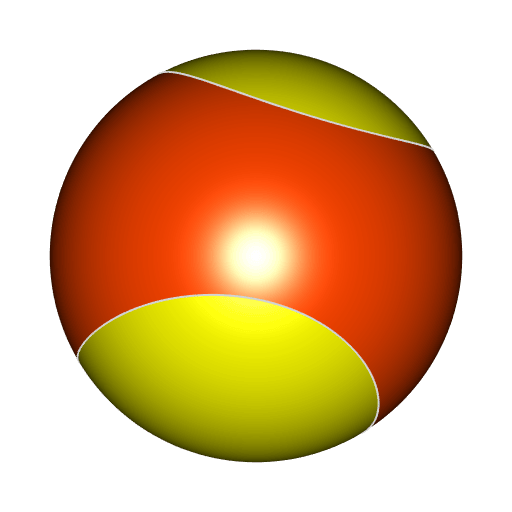# Drawing a tennis ball

Posted on August 10, 2023 by Stéphane Laurent

Short post today. Did you know that the intersection of the Enneper surface with a sphere looks like the seam of a tennis ball? That’s what we shall see.

f <- function(x, y, z) { # Enneper surface: f=0
64*z^9 - 128*z^7 + 64*z^5 - 702*x^2*y^2*z^3 - 18*x^2*y^2*z +
144*(y^2*z^6-x^2*z^6) + 162*(y^4*z^2-x^4*z^2) + 27*(y^6-x^6) +
9*(x^4*z+y^4*z) + 48*(x^2*z^3+y^2*z^3) - 432*(x^2*z^5+y^2*z^5) +
81*(x^4*y^2-x^2*y^4) + 240*(y^2*z^4-x^2*z^4) - 135*(x^4*z^3+y^4*z^3)
}
library(rgl)
smesh <- cgalMeshes::sphereMesh(r = 0.5, iterations = 5L)
mesh1 <- clipMesh3d(smesh, f, greater = TRUE, minVertices = 20000L)
mesh2 <- clipMesh3d(smesh, f, greater = FALSE, minVertices = 20000L)
open3d(windowRect = c(50, 50, 562, 562), zoom = 0.7)
shade3d(mesh1, col = "yellow", polygon_offset = 1)
shade3d(mesh2, col = "orangered", polygon_offset = 1)
b <- getBoundary3d(mesh1, sorted = TRUE, col = "lightgray", lwd = 2)
shade3d(b)